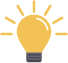### RD Payment BreakupSmart Investment Tip
SIPs have 2x interest rates & can give inflation beating returns as compared to RD.

*Disclaimer: Mutual fund investments are subject to market risks. Please read the offer document carefully before investing

### How to use this RD Calculator?

• Use the slider for Selecting Monthly Amount
• Select the tenure in Months using slider
• Move the slider and select the Interest Rate
• Recalculate your RD anytime by changing the input sliders
• RD Amount will be calculated instantaneously when you move the sliders.

## What is RD?

RD or Recurring Deposits are an investment tool which allows investors to make regular monthly payments and save money for the long term. Investors can choose the tenure of the deposit and the minimum monthly payment they wish to make according to their convenience. RD schemes are generally more flexible than FD schemes and are generally preferred by those who want to start an account for the purpose of saving money and building a rainy-day fund.

## How is RD interest calculated?

Interest on RD is compounded quarterly, in most banks. The formula for this is :

M = R[(1+i)^n-1]/(1-(1+i)^(-1/3) )

In this,

M = Maturity Value

R = Monthly Installment

n = Number of quarters

I = Rate of interest/400

So, if you invest in RD and put in Rs. 5,000 per month for a year, at the interest rate of 8%, your total value will be calculated as:

R = 5000
n = 4 (one year has four quarters)
I = 8.00/400

M = Rs. 62, 647 in one year

## Benefits of RD

• RD can be used as collateral for taking loans. You can take up to 80-90% loans on your RD amount
• Premature withdrawals are allowed under RD. However, this may come with a small penalty
• RD schemes allow for higher rate of interest (usually 0.5% more) for senior citizens
• Minors can also open RD accounts under the supervision and guardianship of their parents
• The tenure is also flexible and you can choose from 7 days to 10 years depending on your convenience
• RD schemes allow you to save money on a regular basis and the minimum deposit amount can be as low as RS. 10

## Tax Benefits on RD

• Similar to other personal tax-saving and investment instruments, Recurring Deposit schemes also attract taxes. A TDS of 10% is deducted on the returns accrued from a RD, if the total interest exceeds Rs. 10,000 in a single financial year.
• Compare this to the SIP scheme and you can see that SIPs are more beneficial for the long term. Since long-term gains from equity are tax free, any SIP which invests in ELSS (Equity Linked Mutual Funds) is also tax free after one year.

## RD Calculator FAQs

• Is RD better than FD?
You must decide on your investment in a fixed deposit vs a recurring deposit depending on the amount you have to invest. For instance, if you have a lump sum amount, you can invest in FDs. However, recurring deposits allow you to invest small amounts regularly and accumulate a sizeable corpus with time.
• Bank FDs are better than recurring deposits for a lump sum investment. Moreover, you can choose the cumulative FD to earn a higher return in the long run.
• Recurring deposits are suitable for investors looking to make small deposits over time.

• What is the maturity amount in RD?
You get the RD maturity amount at the end of the RD tenure. It depends on the duration you have invested your money in the recurring deposit.

Suppose you have deposited Rs 10,000 per month for ten years in an RD account at an interest rate of 8%. Let’s calculate the RD maturity amount.

M = R[(1+i)^n-1]/(1-(1+i)^(-1/3) )

M = Maturity Value
R = Monthly Installment
n = Number of quarters
I = Rate of interest / 400

R = 10,000
n = 4 x 10
i = 8 / 400 = 0.02

M = 10000 x [((1+0.02)^40)-1]/(1-((1+0.02)^(-1/3)))
M = 18,36,167.13.